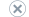# Microsoft Logistic Regression Algorithm

Applies to:SQL Server 2019 and earlier Analysis ServicesAzure Analysis ServicesPower BI Premium

Important

Data mining was deprecated in SQL Server 2017 Analysis Services and now discontinued in SQL Server 2022 Analysis Services. Documentation is not updated for deprecated and discontinued features. To learn more, see Analysis Services backward compatibility.

Logistic regression is a well-known statistical technique that is used for modeling binary outcomes.

There are various implementations of logistic regression in statistics research, using different learning techniques. The Microsoft Logistic Regression algorithm has been implemented by using a variation of the Microsoft Neural Network algorithm. This algorithm shares many of the qualities of neural networks but is easier to train.

One advantage of logistic regression is that the algorithm is highly flexible, taking any kind of input, and supports several different analytical tasks:

• Use demographics to make predictions about outcomes, such as risk for a certain disease.

• Explore and weight the factors that contribute to a result. For example, find the factors that influence customers to make a repeat visit to a store.

• Classify documents, e-mail, or other objects that have many attributes.

## Example

Consider a group of people who share similar demographic information and who buy products from the Adventure Works company. By modeling the data to relate to a specific outcome, such as purchase of a target product, you can see how the demographic information contributes to someone's likelihood of buying the target product.

## How the Algorithm Works

Logistic regression is a well-known statistical method for determining the contribution of multiple factors to a pair of outcomes. The Microsoft implementation uses a modified neural network to model the relationships between inputs and outputs. The effect of each input on the output is measured, and the various inputs are weighted in the finished model. The name logistic regression comes from the fact that the data curve is compressed by using a logistic transformation, to minimize the effect of extreme values. For more information about the implementation, and how to customize the algorithm, see Microsoft Logistic Regression Algorithm Technical Reference.

## Data Required for Logistic Regression Models

When you prepare data for use in training a logistic regression model, you should understand the requirements for the particular algorithm, including how much data is needed, and how the data is used.

The requirements for a logistic regression model are as follows:

A single key column Each model must contain one numeric or text column that uniquely identifies each record. Compound keys are not allowed.

Input columns Each model must contain at least one input column that contains the values that are used as factors in analysis. You can have as many input columns as you want, but depending on the number of values in each column, the addition of extra columns can increase the time it takes to train the model.

At least one predictable column The model must contain at least one predictable column of any data type, including continuous numeric data. The values of the predictable column can also be treated as inputs to the model, or you can specify that it be used for prediction only. Nested tables are not allowed for predictable columns, but can be used as inputs.

For more detailed information about the content types and data types supported for logistic regression models, see the Requirements section of Microsoft Logistic Regression Algorithm Technical Reference.

## Viewing a Logistic Regression Model

To explore the model, you can use the Microsoft Neural Network Viewer, or the Microsoft Generic Content Tree Viewer.

When you view the model by using the Microsoft Neural Network Viewer, Analysis Services shows you the factors that contribute to a particular outcome, ranked by their importance. You can choose an attribute and values to compare. For more information, see Browse a Model Using the Microsoft Neural Network Viewer.

If you want to know more, you can browse the model details by using the Microsoft Generic Content Tree Viewer. The model content for a logistic regression model includes a marginal node that shows you the all the inputs used for the model, and subnetworks for the predictable attributes. For more information, see Mining Model Content for Logistic Regression Models (Analysis Services - Data Mining).

## Creating Predictions

After the model has been trained, you can create queries against the model content to get the regression coefficients and other details, or you can use the model to make predictions.

## Remarks

• Does not support drillthrough. This is because the structure of nodes in the mining model does not necessarily correspond directly to the underlying data.

• Does not support the creation of data mining dimensions.

• Supports the use of OLAP mining models.

• Does not support the use of Predictive Model Markup Language (PMML) to create mining models.